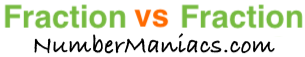1/2 vs 1/4People compare 1/2 vs 1/4 drywall, 1/2 vs 1/4 pipe, 1/2 vs 1/4 cup, 1/2 vs 1/4 ton, 1/2 vs 1/4 drill bit, 1/2 vs 1/4 poker, 1/2 vs 1/4 sump pump, 1/2 vs 1/4 garden hose, and many other items.

Is 1/2 greater than 1/4? Is 1/2 less than 1/4? What is the difference between 1/2 and 1/4? These and other questions are answered below so you can get a better perspective of 1/2 vs 1/4.

1/2 vs 1/4 Fractional Comparisons
1/2 is more than 1/4
The difference between 1/2 and 1/4 is 1/4
1/2 is 1/4 more than 1/4

1/2 vs 1/4 Decimal Comparisons
1/2 vs 1/4 as a decimal is 0.5 vs 0.25
The difference between 1/2 and 1/4 is 0.25
1/2 is 0.25 more than 1/4

1/2 vs 1/4 Percent Comparisons
1/2 vs 1/4 as a percent is 50% vs 25%
1/2 is 100 percent more than 1/4

Fraction vs Fraction
Submit another set of fractions that we can compare for you!

/
vs
/

The math behind the numbers
Calculations on this page are rounded to the nearest thousandth if necessary. Below is the math we used to analyze 1/2 vs 1/4:

To compare the size of 1/2 vs 1/4, we simply subtracted 1/4 from 1/2:

1/2 - 1/4 = |1/4|

To find 1/2 vs 1/4 as a decimal, we divided the numerator by the denominator for each fraction.

1 ÷ 2 = 0.5
1 ÷ 4 = 0.25

To find 1/2 vs 1/4 as a percent, we divided the numerator by the denominator and then multiplied the quotient by 100 for each fraction.

(1 ÷ 2) x 100 = 50%
(1 ÷ 4) x 100 = 25%

We found that 1/2 is 100 percent more than 1/4 by dividing the difference between the two fractions by the second fraction and then multiplied it by 100:

((1/4)/(1/4))×100 = 100%

That's it folks. We hope this page gave you a better understanding of 1/2 vs 1/4 with our explanations and conclusions.

1/2 vs 1/5
Here are the next two fractions we have compared and analyzed.